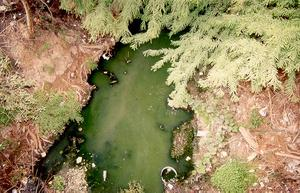online_do:   Dissolved oxygen (DO) sag curveSs = f(T)           L = Lr/n O0 = [Qs/(Qs + Qe)] Os Oj+1 = Oj + L (ko/v) (Ss - Oj) - L (kd/v) Du e-(kd/v)xj+1

INPUT DATA:

Select:

 SI units (metric) U.S. Customary units

[Choose SI or U.S. Customary units].   [Default: SI units; program resets to default units].

Stream (optional):

Stream discharge Qs (m3/s) [ft3/s]:

Water temperature T (oC) [oF]:

[Saturation dissolved oxygen is at zero salinity and mean sea level].

Dissolved oxygen (upstream) Os (mg/L):

Effluent discharge Qe (L/s) [ft3/s]:

Effluent biochemical oxygen demand De (mg/L):

Stream velocity v [m/s] [ft/s]:

Deoxygenation constant kd (day-1):

Oxygenation constant ko (day-1):

Total reach length Lr (km) [mi]:

Number of space steps n:
(nmax = 200)
[ Leave blank to choose default n = 10 ]

OUTPUT:

 Spaceintervalj Distance(km) DO(this model)(mg/L) DO(Streeter-Phelps)(mg/L) DOdifference(mg/L)

Your request was processed at  04:29:24 am on June 27th, 2022